# RD Sharma Solutions for Class 6 Maths Chapter 23: Data Handling - III (Bar Graphs) Exercise 23.2

In this exercise, students gain knowledge about the important steps to be followed for the construction of Bar Graphs. It is a very simple method which can be used in order to represent the given numerical data. The solutions are prepared in a comprehensive manner to make the concepts simpler for the students while studying. To know more about the concepts which are covered under this exercise, students can download RD Sharma Solutions Class 6 Maths Chapter 23 Data Handling – III (Bar Graphs) Exercise 23.2 PDF from the links which are provided here.

## RD Sharma Solutions for Class 6 Maths Chapter 23: Data Handling – III (Bar Graphs) Exercise 23.2 Download PDF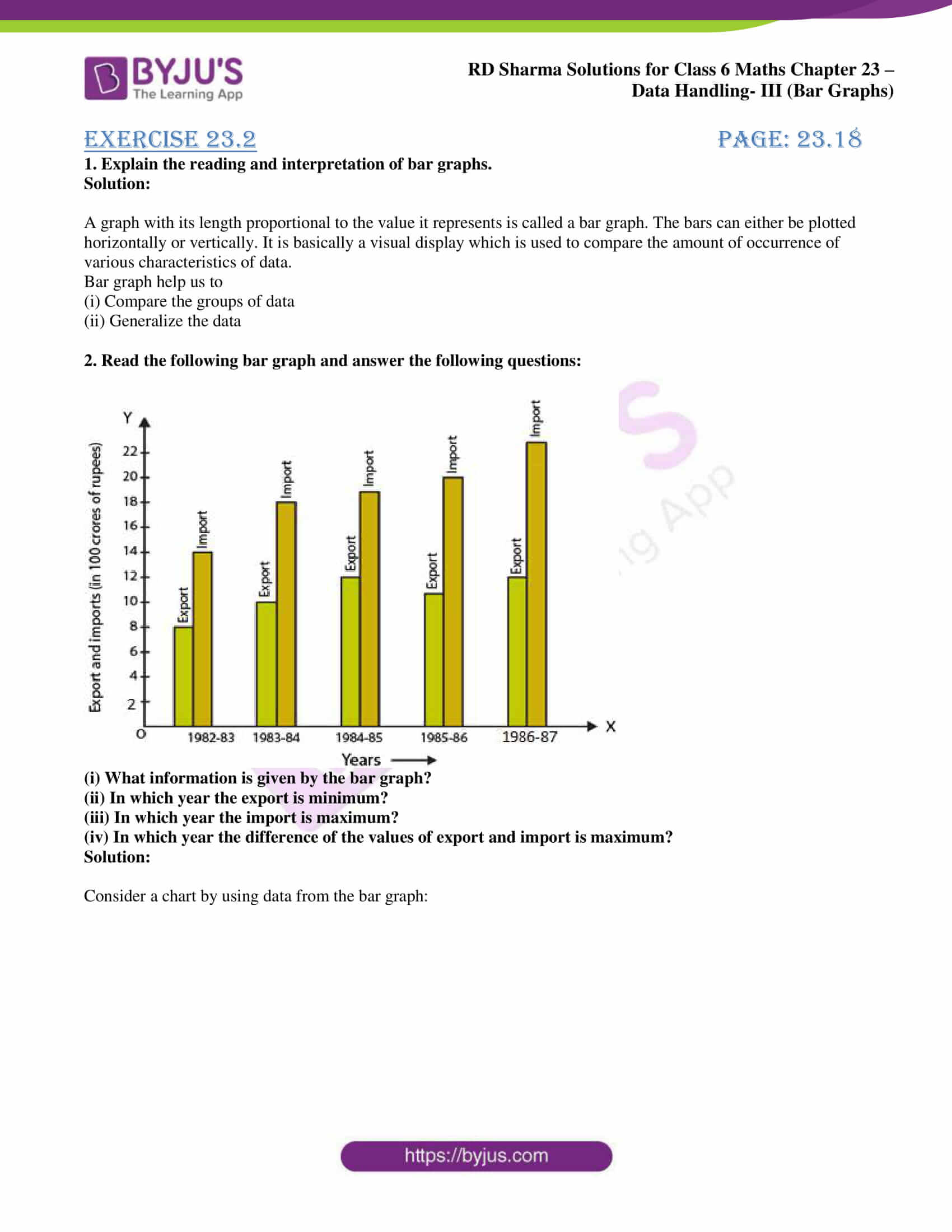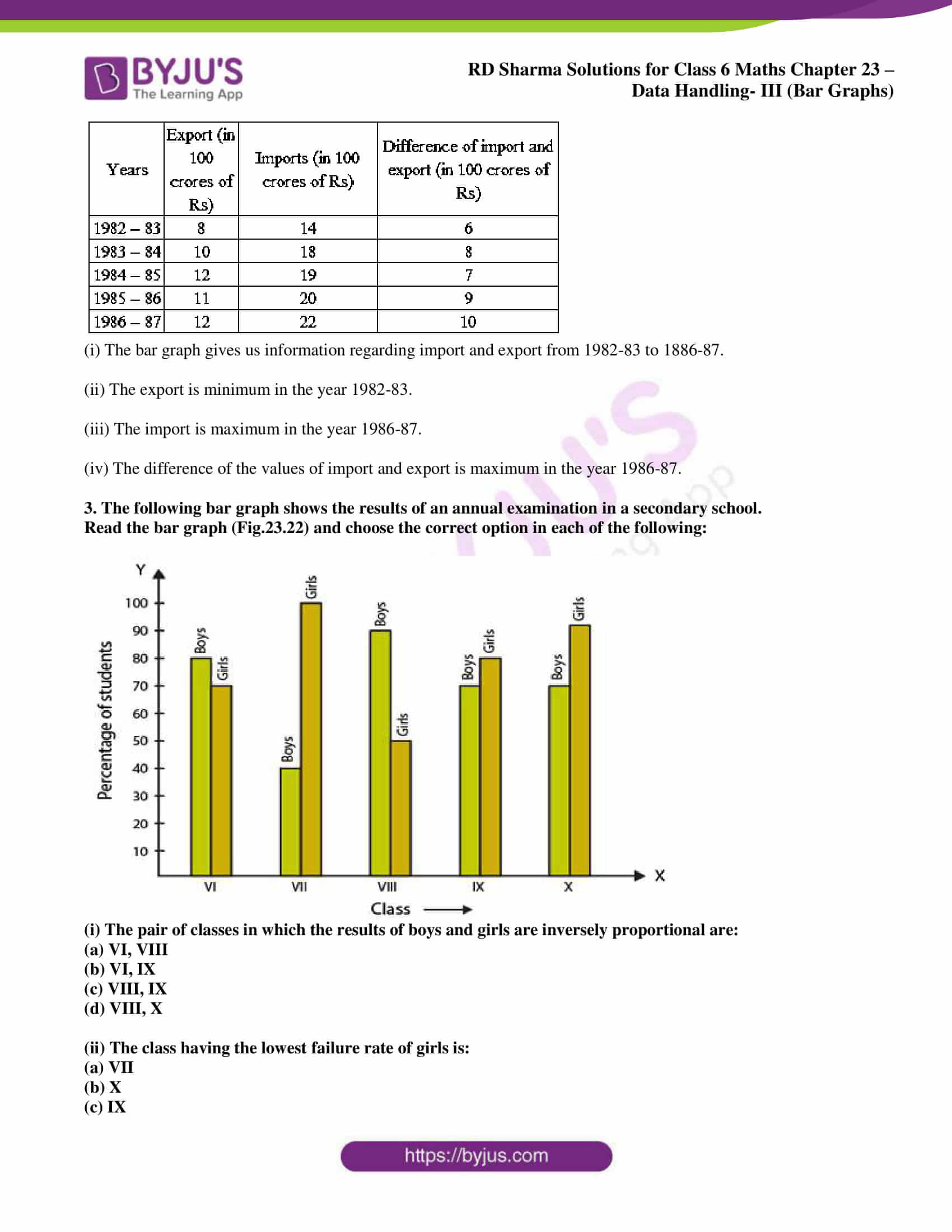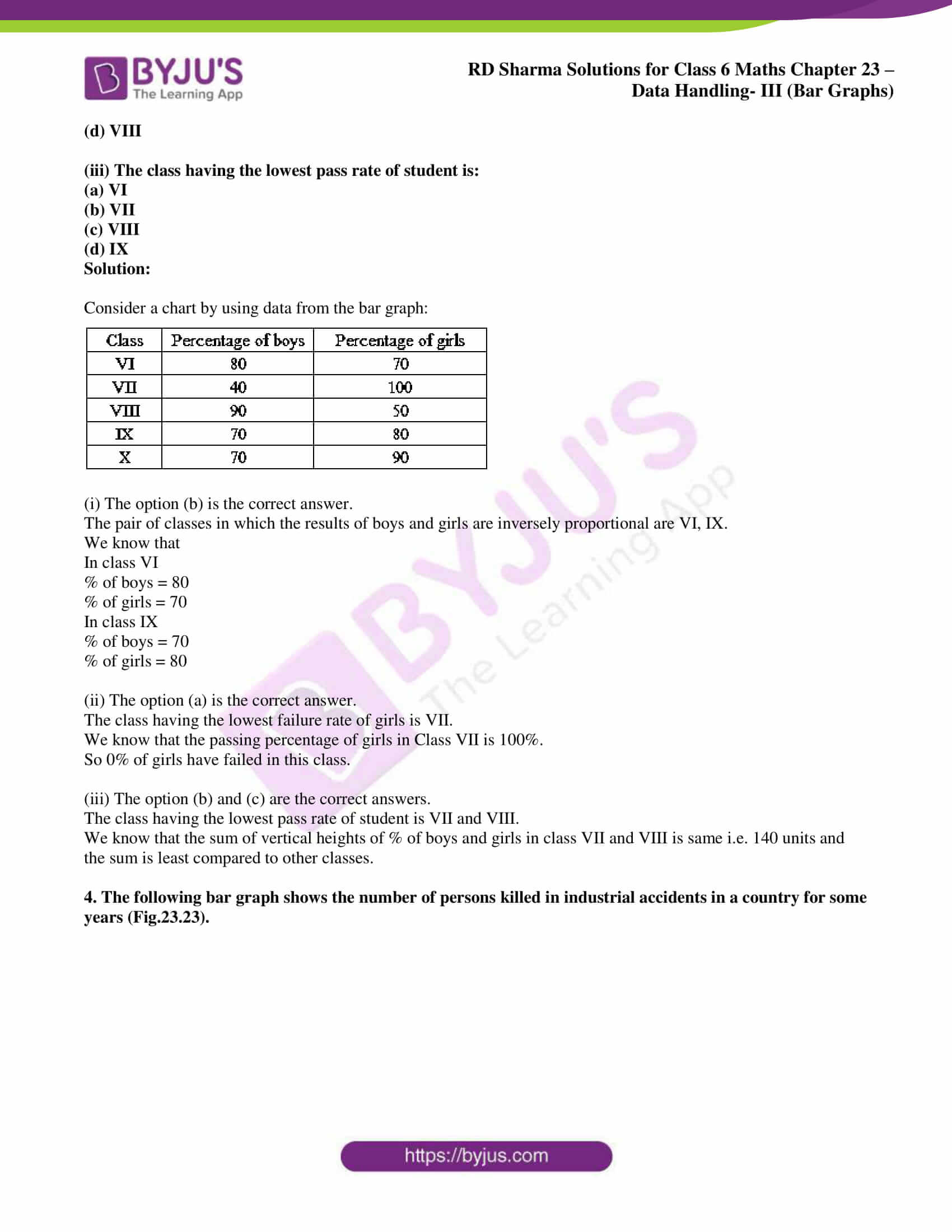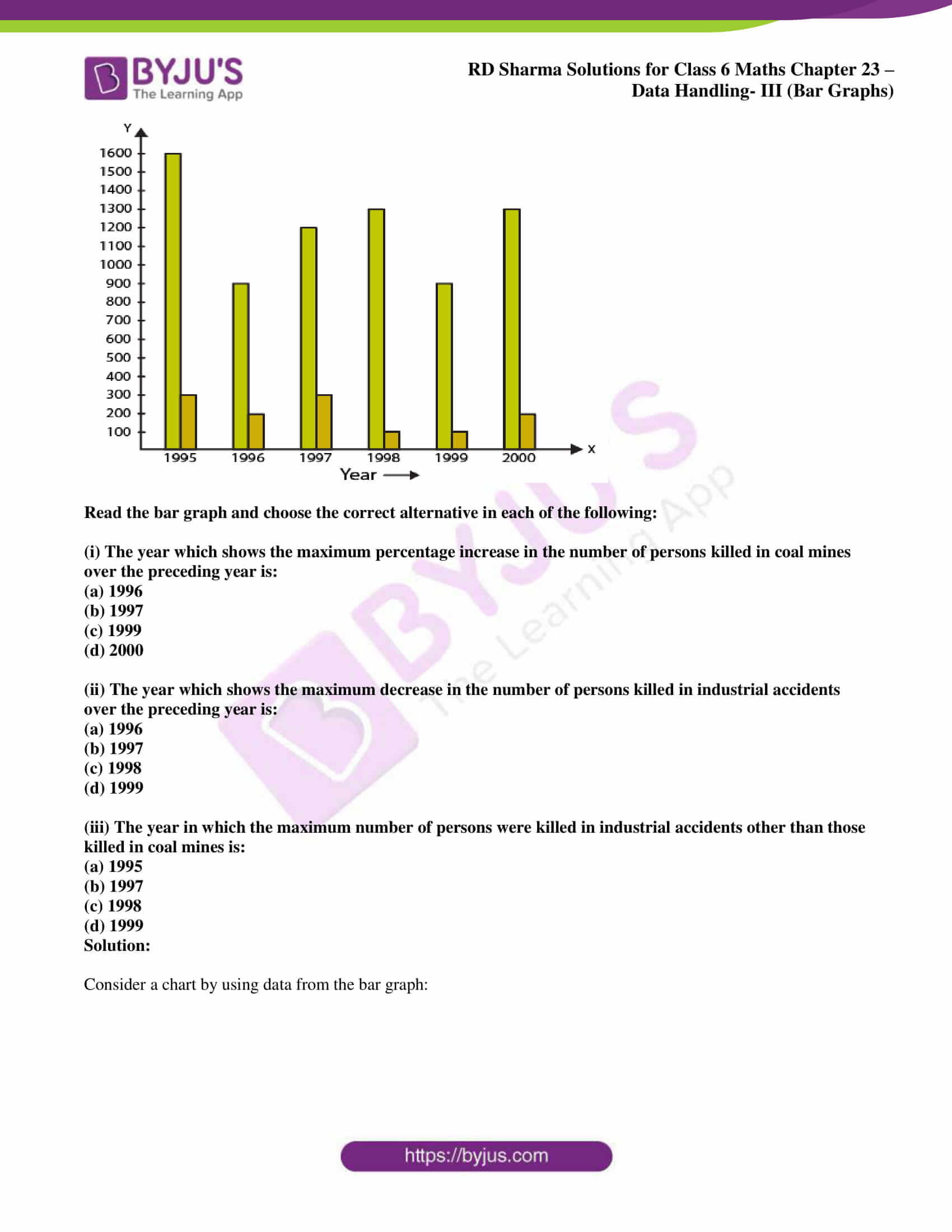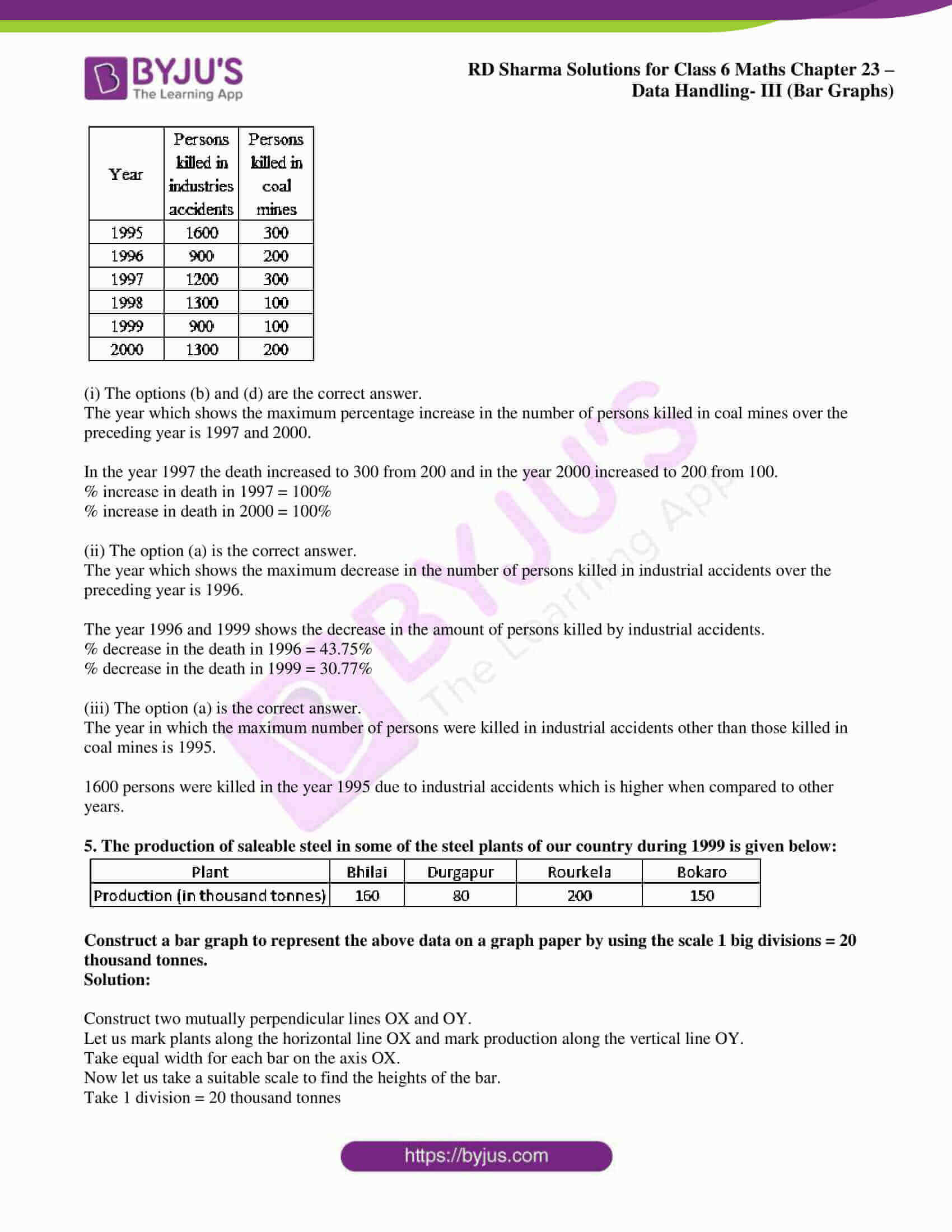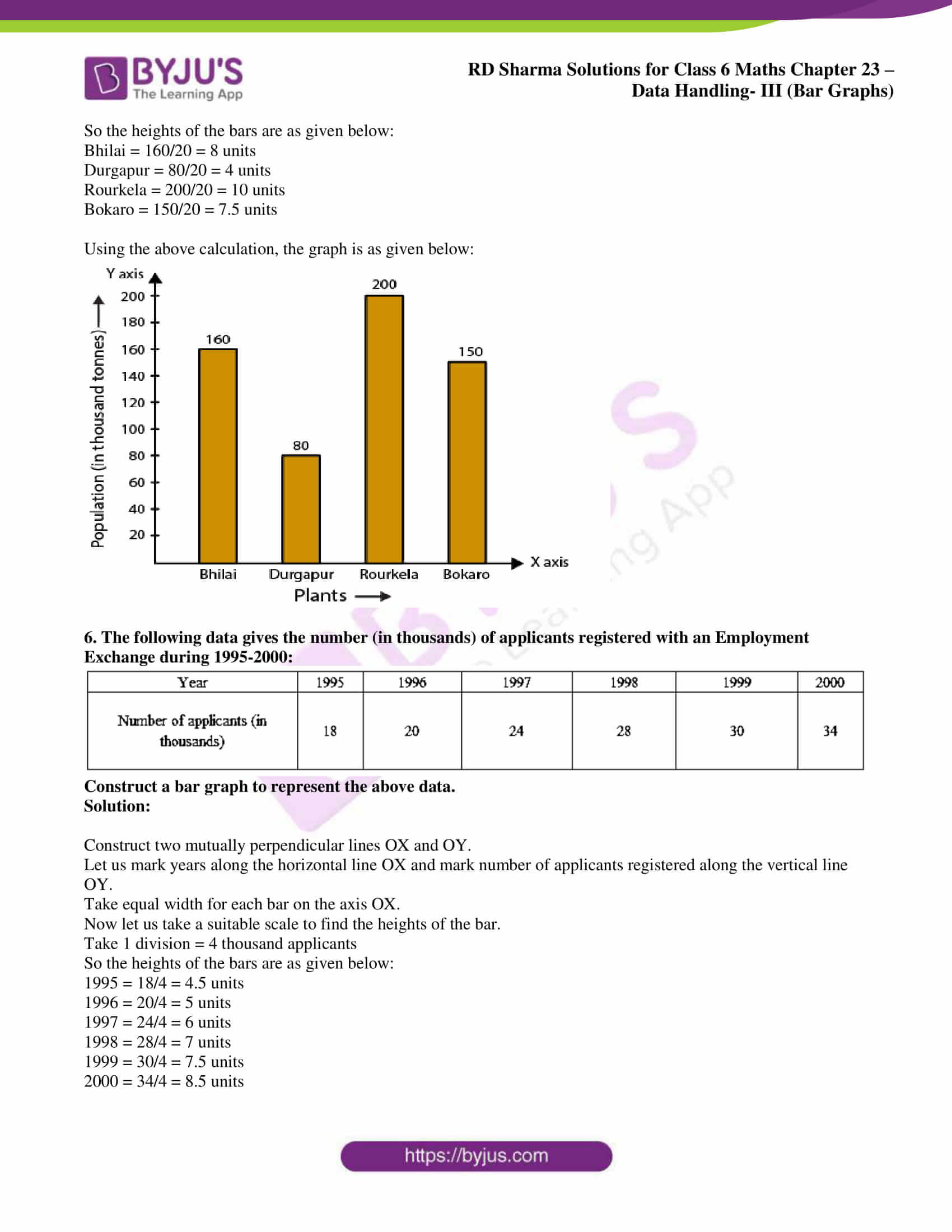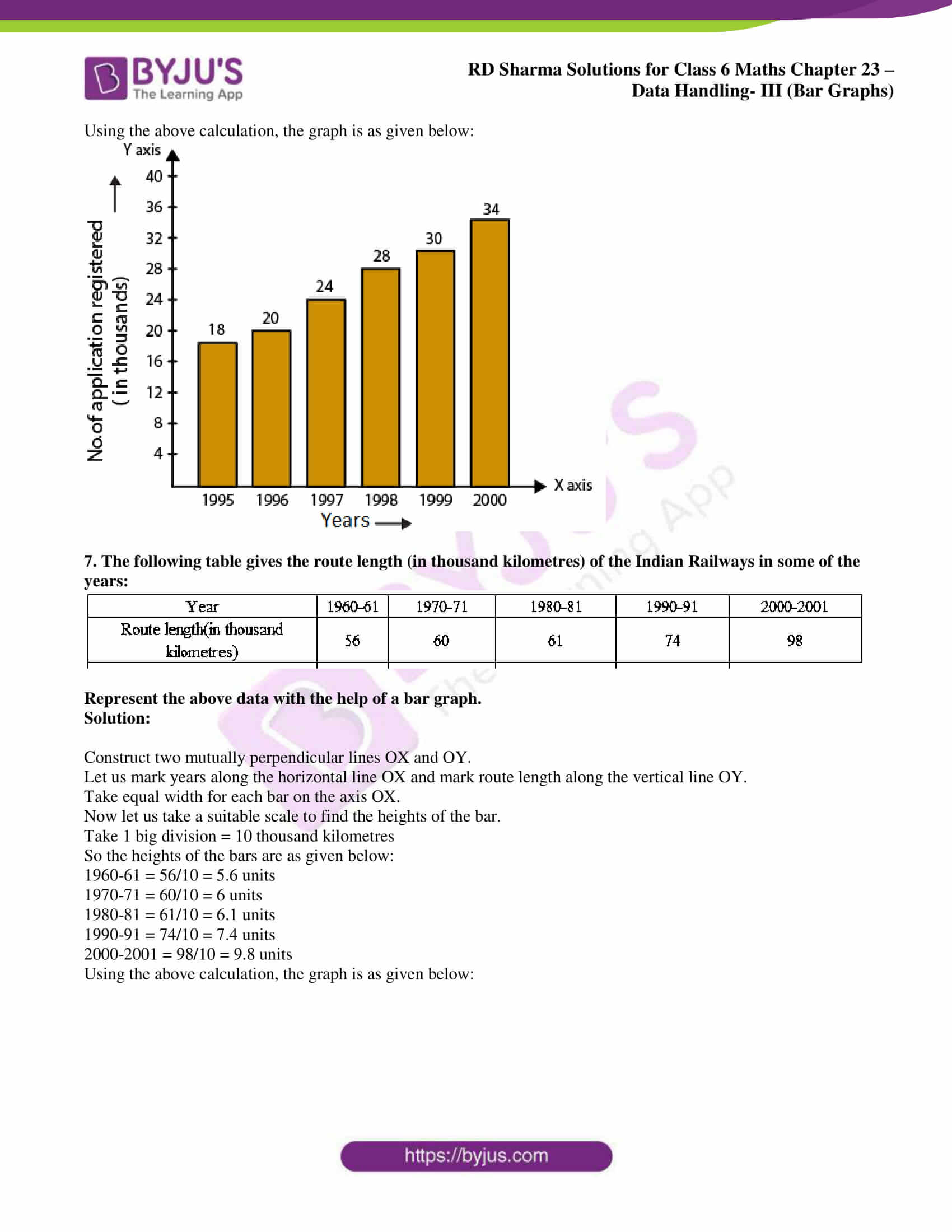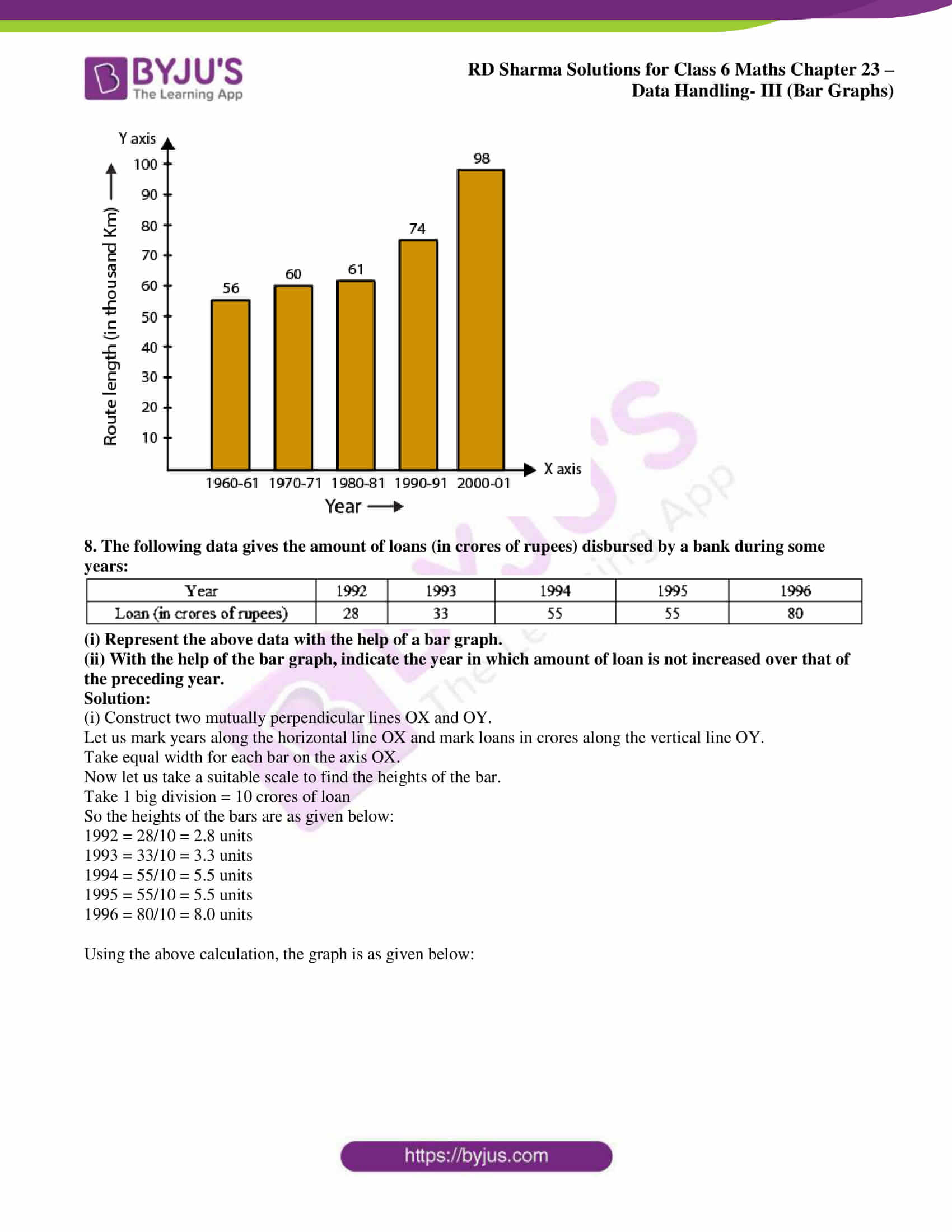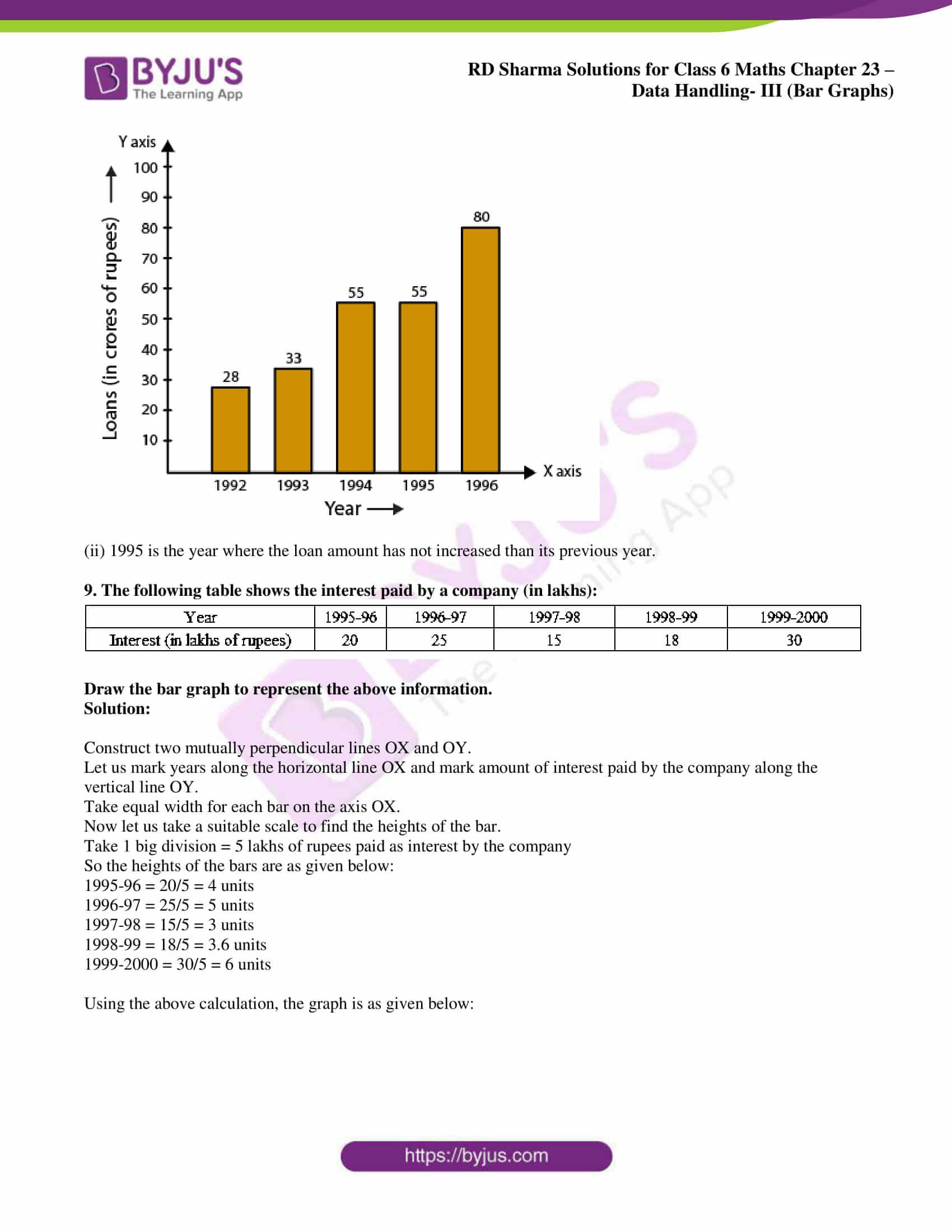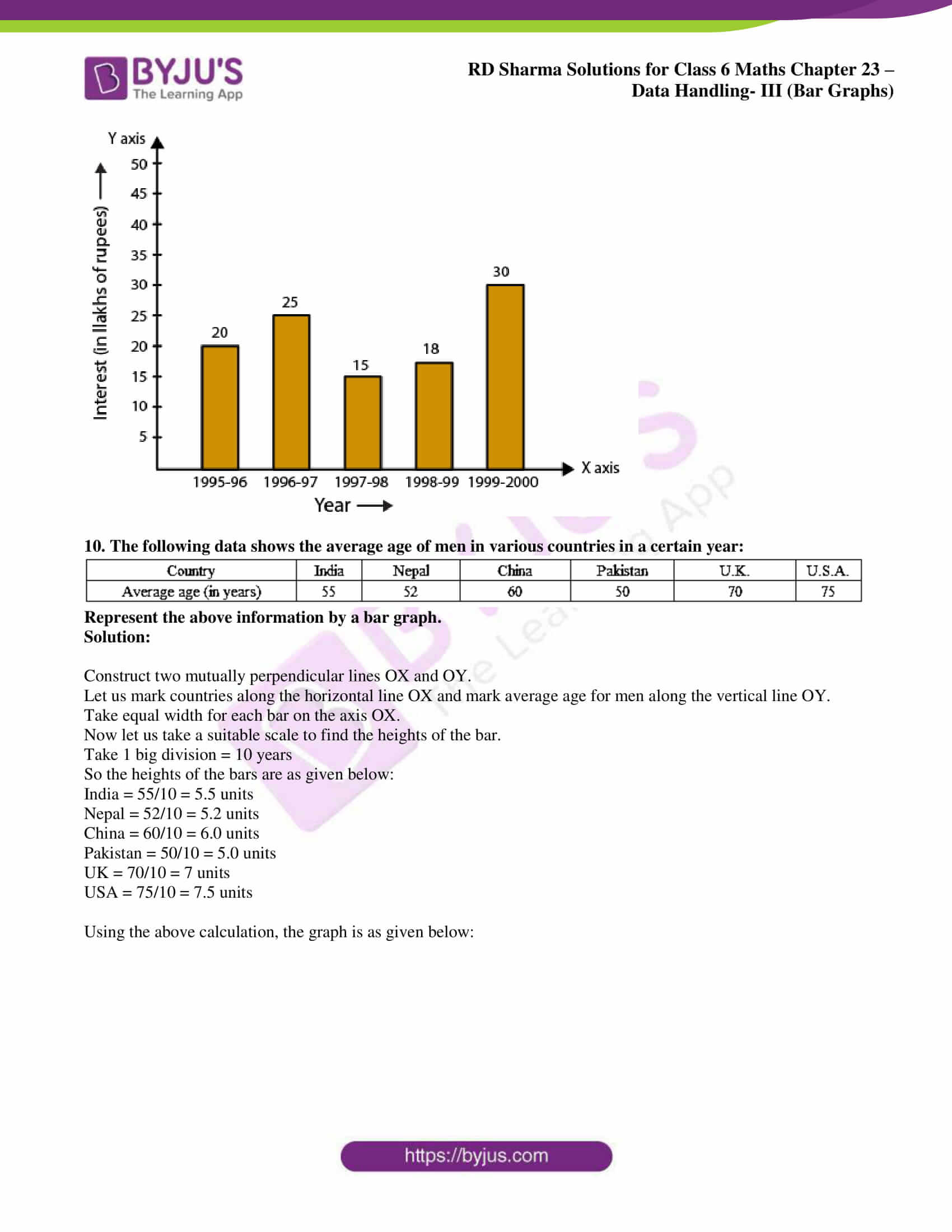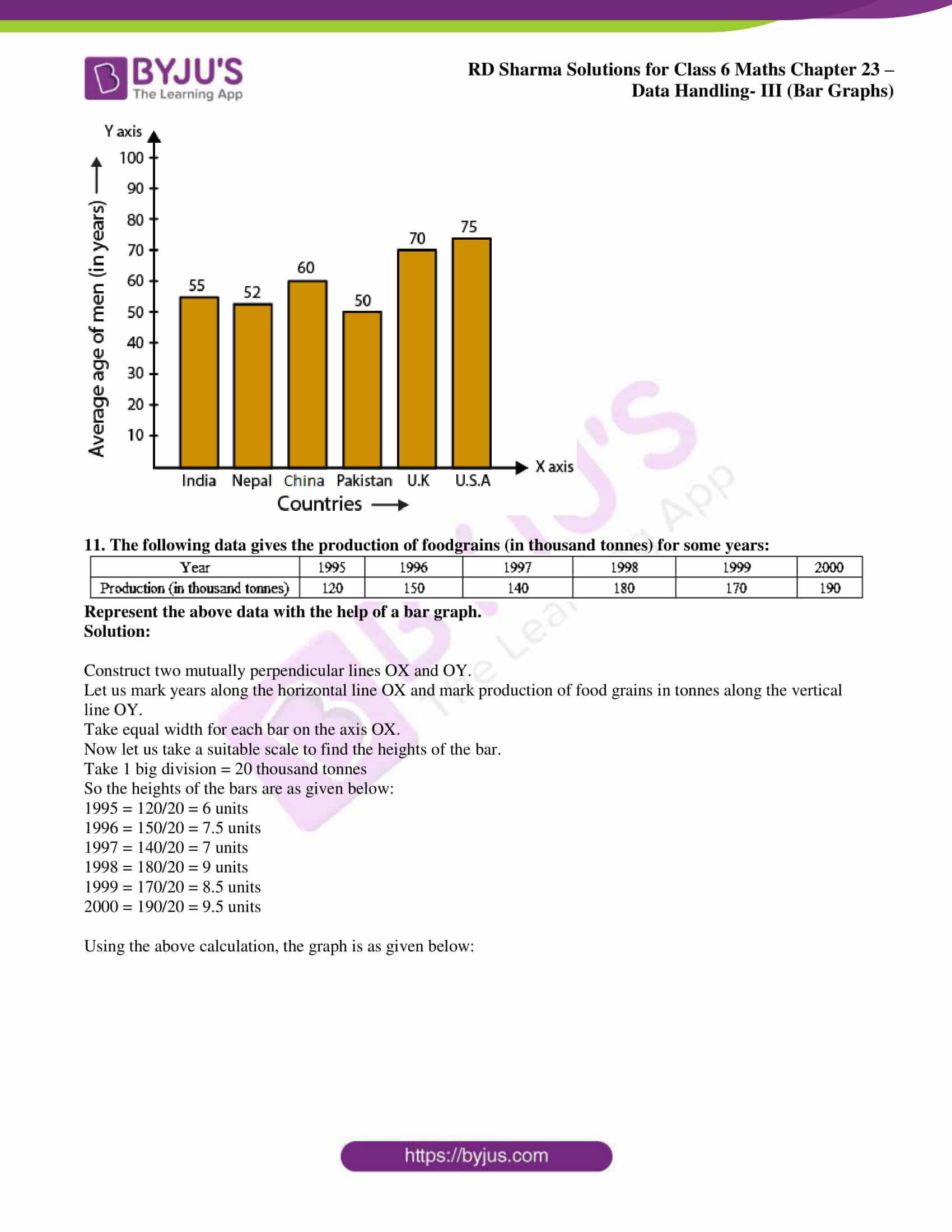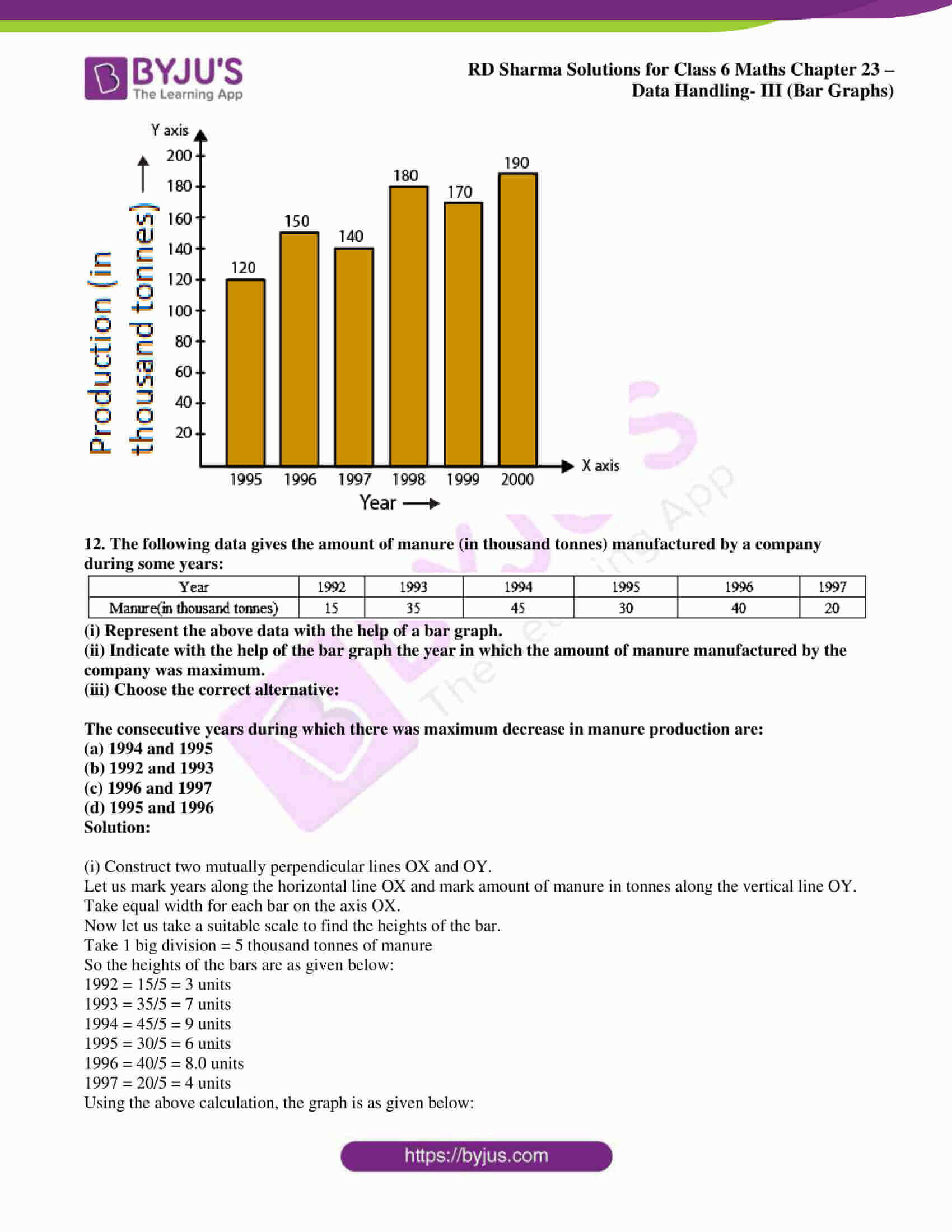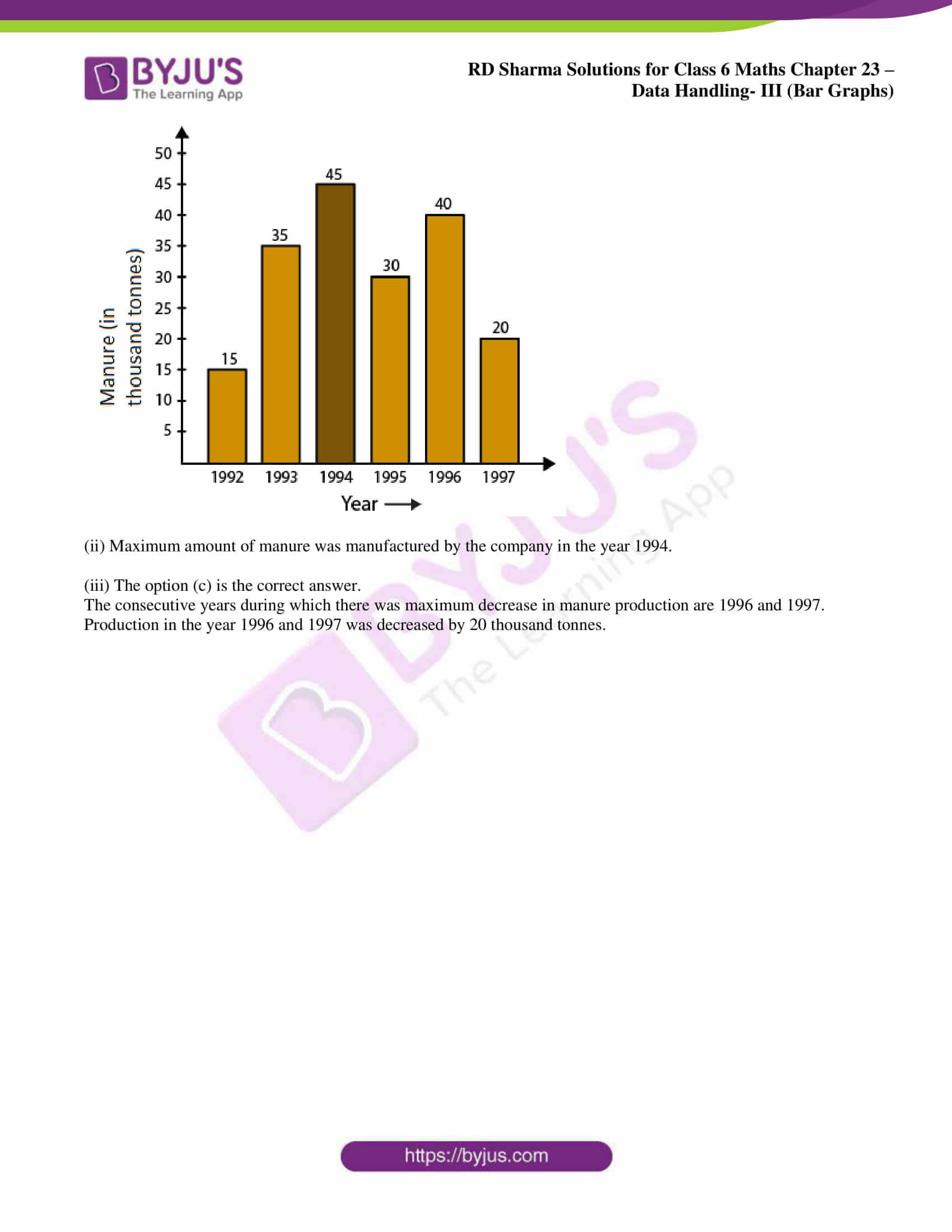### Exercise 23.2 page: 23.18

1. Explain the reading and interpretation of bar graphs.

Solution:

A graph with its length proportional to the value it represents is called a bar graph. The bars can either be plotted horizontally or vertically. It is basically a visual display which is used to compare the amount of occurrence of various characteristics of data.

Bar graph help us to

(i) Compare the groups of data

(ii) Generalize the data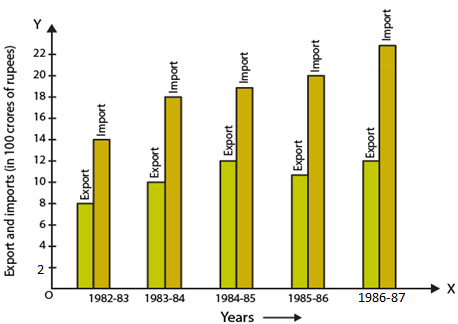(i) What information is given by the bar graph?
(ii) In which year the export is minimum?
(iii) In which year the import is maximum?
(iv) In which year the difference of the values of export and import is maximum?

Solution:

Consider a chart by using data from the bar graph: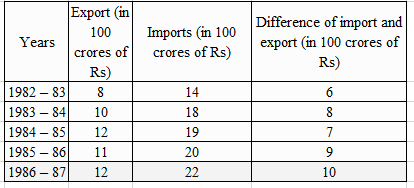(i) The bar graph gives us information regarding import and export from 1982-83 to 1886-87.

(ii) The export is minimum in the year 1982-83.

(iii) The import is maximum in the year 1986-87.

(iv) The difference of the values of import and export is maximum in the year 1986-87.

3. The following bar graph shows the results of an annual examination in a secondary school.
Read the bar graph (Fig.23.22) and choose the correct option in each of the following: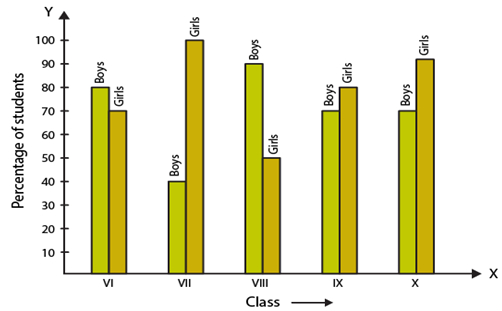(i) The pair of classes in which the results of boys and girls are inversely proportional are:
(a) VI, VIII
(b) VI, IX
(c) VIII, IX
(d) VIII, X

(ii) The class having the lowest failure rate of girls is:
(a) VII
(b) X
(c) IX
(d) VIII

(iii) The class having the lowest pass rate of student is:
(a) VI
(b) VII
(c) VIII
(d) IX

Solution:

Consider a chart by using data from the bar graph: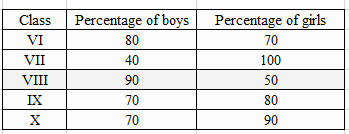(i) The option (b) is the correct answer.

The pair of classes in which the results of boys and girls are inversely proportional are VI, IX.

We know that

In class VI

% of boys = 80

% of girls = 70

In class IX

% of boys = 70

% of girls = 80

(ii) The option (a) is the correct answer.

The class having the lowest failure rate of girls is VII.

We know that the passing percentage of girls in Class VII is 100%.

So 0% of girls have failed in this class.

(iii) The option (b) and (c) are the correct answers.

The class having the lowest pass rate of student is VII and VIII.

We know that the sum of vertical heights of % of boys and girls in class VII and VIII is same i.e. 140 units and the sum is least compared to other classes.

4. The following bar graph shows the number of persons killed in industrial accidents in a country for some years (Fig.23.23).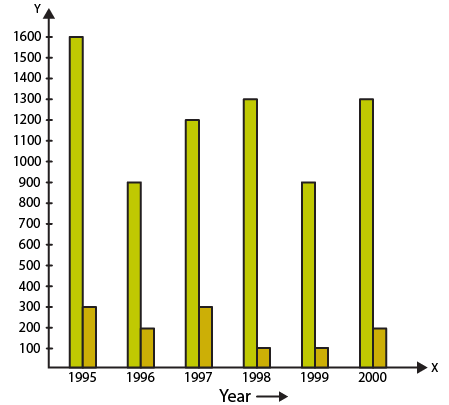Read the bar graph and choose the correct alternative in each of the following:

(i) The year which shows the maximum percentage increase in the number of persons killed in coal mines over the preceding year is:
(a) 1996
(b) 1997
(c) 1999
(d) 2000

(ii) The year which shows the maximum decrease in the number of persons killed in industrial accidents over the preceding year is:
(a) 1996
(b) 1997
(c) 1998
(d) 1999

(iii) The year in which the maximum number of persons were killed in industrial accidents other than those killed in coal mines is:
(a) 1995
(b) 1997
(c) 1998
(d) 1999

Solution:

Consider a chart by using data from the bar graph: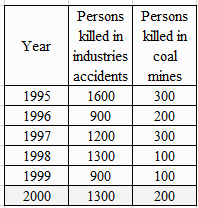(i) The options (b) and (d) are the correct answer.

The year which shows the maximum percentage increase in the number of persons killed in coal mines over the preceding year is 1997 and 2000.

In the year 1997 the death increased to 300 from 200 and in the year 2000 increased to 200 from 100.

% increase in death in 1997 = 100%

% increase in death in 2000 = 100%

(ii) The option (a) is the correct answer.

The year which shows the maximum decrease in the number of persons killed in industrial accidents over the preceding year is 1996.

The year 1996 and 1999 shows the decrease in the amount of persons killed by industrial accidents.

% decrease in the death in 1996 = 43.75%

% decrease in the death in 1999 = 30.77%

(iii) The option (a) is the correct answer.

The year in which the maximum number of persons were killed in industrial accidents other than those killed in coal mines is 1995.

1600 persons were killed in the year 1995 due to industrial accidents which is higher when compared to other years.

5. The production of saleable steel in some of the steel plants of our country during 1999 is given below: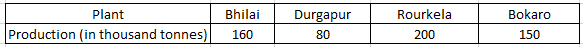Construct a bar graph to represent the above data on a graph paper by using the scale 1 big divisions = 20 thousand tonnes.

Solution:

Construct two mutually perpendicular lines OX and OY.

Let us mark plants along the horizontal line OX and mark production along the vertical line OY.

Take equal width for each bar on the axis OX.

Now let us take a suitable scale to find the heights of the bar.

Take 1 division = 20 thousand tonnes

So the heights of the bars are as given below:

Bhilai = 160/20 = 8 units

Durgapur = 80/20 = 4 units

Rourkela = 200/20 = 10 units

Bokaro = 150/20 = 7.5 units

Using the above calculation, the graph is as given below: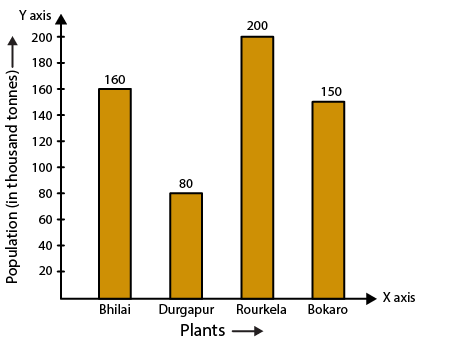6. The following data gives the number (in thousands) of applicants registered with an Employment Exchange during 1995-2000: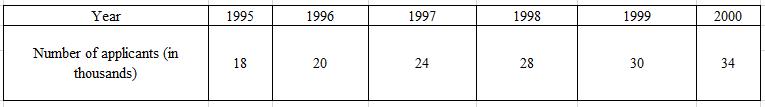Construct a bar graph to represent the above data.

Solution:

Construct two mutually perpendicular lines OX and OY.

Let us mark years along the horizontal line OX and mark number of applicants registered along the vertical line OY.

Take equal width for each bar on the axis OX.

Now let us take a suitable scale to find the heights of the bar.

Take 1 division = 4 thousand applicants

So the heights of the bars are as given below:

1995 = 18/4 = 4.5 units

1996 = 20/4 = 5 units

1997 = 24/4 = 6 units

1998 = 28/4 = 7 units

1999 = 30/4 = 7.5 units

2000 = 34/4 = 8.5 units

Using the above calculation, the graph is as given below: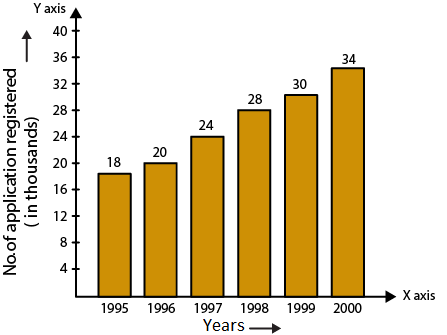7. The following table gives the route length (in thousand kilometres) of the Indian Railways in some of the years: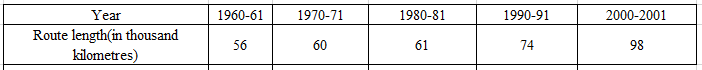Represent the above data with the help of a bar graph.

Solution:

Construct two mutually perpendicular lines OX and OY.

Let us mark years along the horizontal line OX and mark route length along the vertical line OY.

Take equal width for each bar on the axis OX.

Now let us take a suitable scale to find the heights of the bar.

Take 1 big division = 10 thousand kilometres

So the heights of the bars are as given below:

1960-61 = 56/10 = 5.6 units

1970-71 = 60/10 = 6 units

1980-81 = 61/10 = 6.1 units

1990-91 = 74/10 = 7.4 units

2000-2001 = 98/10 = 9.8 units

Using the above calculation, the graph is as given below: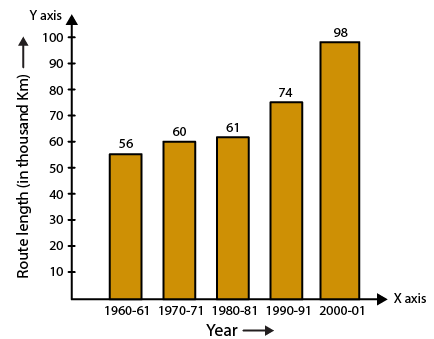8. The following data gives the amount of loans (in crores of rupees) disbursed by a bank during some years: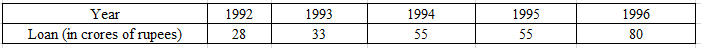(i) Represent the above data with the help of a bar graph.
(ii) With the help of the bar graph, indicate the year in which amount of loan is not increased over that of the preceding year.

Solution:

(i) Construct two mutually perpendicular lines OX and OY.

Let us mark years along the horizontal line OX and mark loans in crores along the vertical line OY.

Take equal width for each bar on the axis OX.

Now let us take a suitable scale to find the heights of the bar.

Take 1 big division = 10 crores of loan

So the heights of the bars are as given below:

1992 = 28/10 = 2.8 units

1993 = 33/10 = 3.3 units

1994 = 55/10 = 5.5 units

1995 = 55/10 = 5.5 units

1996 = 80/10 = 8.0 units

Using the above calculation, the graph is as given below: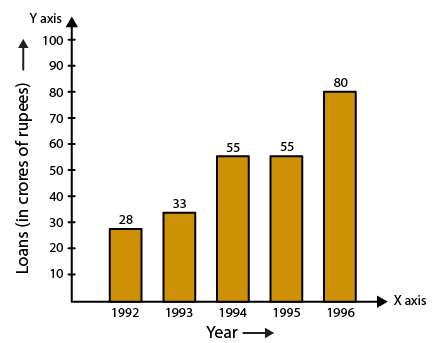(ii) 1995 is the year where the loan amount has not increased than its previous year.

9. The following table shows the interest paid by a company (in lakhs):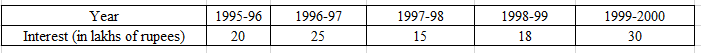Draw the bar graph to represent the above information.

Solution:

Construct two mutually perpendicular lines OX and OY.

Let us mark years along the horizontal line OX and mark amount of interest paid by the company along the vertical line OY.

Take equal width for each bar on the axis OX.

Now let us take a suitable scale to find the heights of the bar.

Take 1 big division = 5 lakhs of rupees paid as interest by the company

So the heights of the bars are as given below:

1995-96 = 20/5 = 4 units

1996-97 = 25/5 = 5 units

1997-98 = 15/5 = 3 units

1998-99 = 18/5 = 3.6 units

1999-2000 = 30/5 = 6 units

Using the above calculation, the graph is as given below: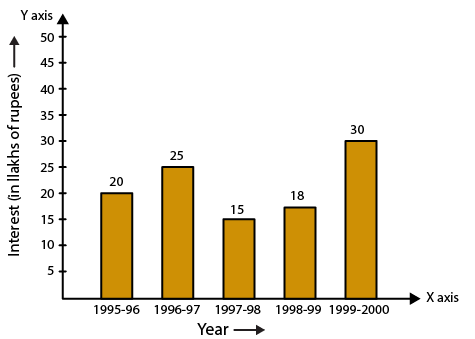10. The following data shows the average age of men in various countries in a certain year: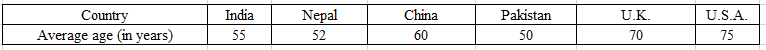Represent the above information by a bar graph.

Solution:

Construct two mutually perpendicular lines OX and OY.

Let us mark countries along the horizontal line OX and mark average age for men along the vertical line OY.

Take equal width for each bar on the axis OX.

Now let us take a suitable scale to find the heights of the bar.

Take 1 big division = 10 years

So the heights of the bars are as given below:

India = 55/10 = 5.5 units

Nepal = 52/10 = 5.2 units

China = 60/10 = 6.0 units

Pakistan = 50/10 = 5.0 units

UK = 70/10 = 7 units

USA = 75/10 = 7.5 units

Using the above calculation, the graph is as given below: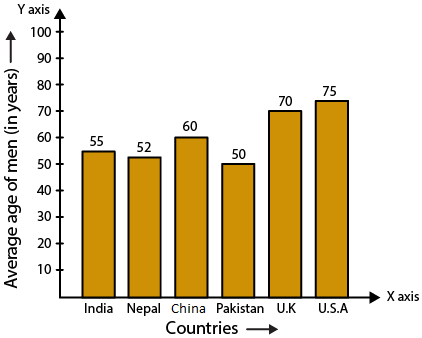11. The following data gives the production of foodgrains (in thousand tonnes) for some years: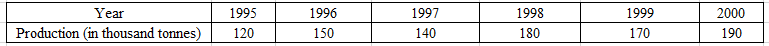Represent the above data with the help of a bar graph.

Solution:

Construct two mutually perpendicular lines OX and OY.

Let us mark years along the horizontal line OX and mark production of food grains in tonnes along the vertical line OY.

Take equal width for each bar on the axis OX.

Now let us take a suitable scale to find the heights of the bar.

Take 1 big division = 20 thousand tonnes

So the heights of the bars are as given below:

1995 = 120/20 = 6 units

1996 = 150/20 = 7.5 units

1997 = 140/20 = 7 units

1998 = 180/20 = 9 units

1999 = 170/20 = 8.5 units

2000 = 190/20 = 9.5 units

Using the above calculation, the graph is as given below: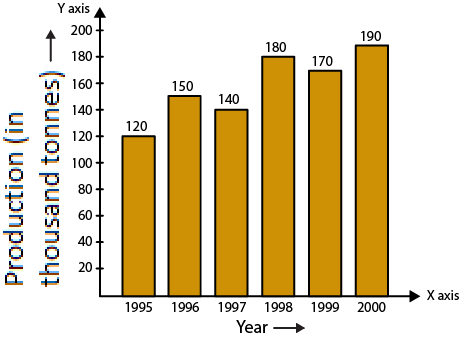12. The following data gives the amount of manure (in thousand tonnes) manufactured by a company during some years: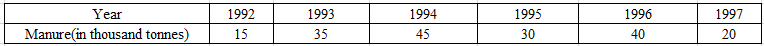(i) Represent the above data with the help of a bar graph.
(ii) Indicate with the help of the bar graph the year in which the amount of manure manufactured by the company was maximum.
(iii) Choose the correct alternative:

The consecutive years during which there was maximum decrease in manure production are:
(a) 1994 and 1995
(b) 1992 and 1993
(c) 1996 and 1997
(d) 1995 and 1996

Solution:

(i) Construct two mutually perpendicular lines OX and OY.

Let us mark years along the horizontal line OX and mark amount of manure in tonnes along the vertical line OY.

Take equal width for each bar on the axis OX.

Now let us take a suitable scale to find the heights of the bar.

Take 1 big division = 5 thousand tonnes of manure

So the heights of the bars are as given below:

1992 = 15/5 = 3 units

1993 = 35/5 = 7 units

1994 = 45/5 = 9 units

1995 = 30/5 = 6 units

1996 = 40/5 = 8.0 units

1997 = 20/5 = 4 units

Using the above calculation, the graph is as given below: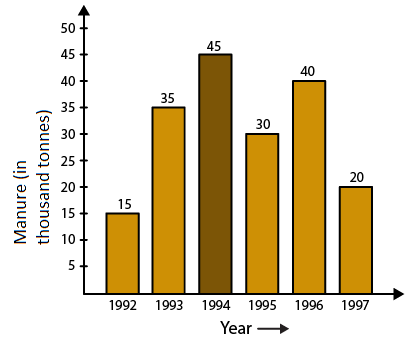(ii) Maximum amount of manure was manufactured by the company in the year 1994.

(iii) The option (c) is the correct answer.

The consecutive years during which there was maximum decrease in manure production are 1996 and 1997.

Production in the year 1996 and 1997 was decreased by 20 thousand tonnes.